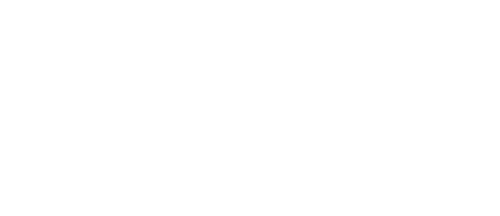Apply Now!# MATH155M - College Algebra with Trigonometry (4-0-4)

This course covers the essentials of numerical algebra, geometry, and trigonometry and is designed for science, engineering, technology, computer science, and mathematics students. It provides a solid preparation for student toward Precalculus and Calculus track. A short review of elementary algebra is followed by an introduction to geometric and trigonometric functions. Applied problems are solved by integrating the above mathematical strategies. The trigonometric functions include ratios in solving right triangles and vector applications, and Law of Sines and Cosines in solving oblique triangles.

Prerequisite: MATH151M with a grade of “C” or better, satisfactory placement test scores, or permission from the Mathematics Program Coordinator, the Department Chair, or a full time mathematics faculty member.